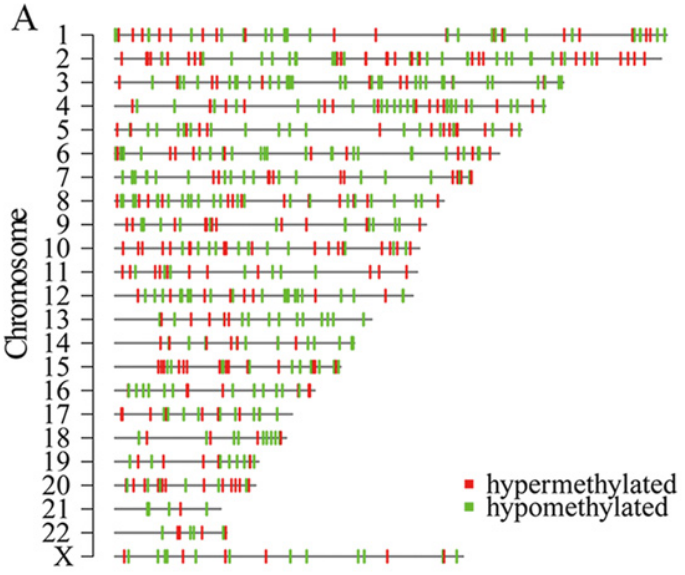Cannot identify software used to make image
2
1
Entering edit mode
2.7 years ago
dec986 ▴ 300

Hello, I'm trying to identify the image used to make this image, I cannot find out, and the author of the paper Figure 2A in DOI:10.1371/journal.pone.0120388 isn't answering his email, does anyone recognize what software was used to make this image?

an edit to BioStars: none of these free image hosting is working

[IMG]http://i65.tinypic.com/fjkf2b.png[/IMG]https://ibb.co/ZSftjb8DMR • 1.0k views
0
Entering edit mode

You should be able to right click the image, copy address and insert it using the image button:and in text it should show up as:

![description of image]

: https://i.postimg.cc/gJ7vztXC/Screenshot-from-2019-02-15-20-41-50.png

6
Entering edit mode
2.7 years ago
bernatgel ★ 3.2k

I don't know the exact software used to create that image but you can reproduce it using karyoploteR.Here's the code. We start creating random regions on the genome with regioneR's createRandomRegionsfunction. We the create the plot, using plot.type=6 with no ideograms. After that we use kpAbline to plot the horizontal black lines and kpPlotRegions to plot the DMR regions in two colors. In total, 6 lines of code + 1 for the legend.

In the karyoploteR tutorial page you can find much more information, including how to add many other data types and how to customize the plot style.

library(karyoploteR)

#Create random regione with regioneR's createRandomRegions
up.dmr <- createRandomRegions(nregions = 40, length.mean = 2e6)
down.dmr <- createRandomRegions(nregions = 40, length.mean = 2e6)

#Initialize the plot
kp <- plotKaryotype(plot.type = 6, ideogram.plotter = NULL)

#Create the black lines
kpAbline(kp, h=0.5)

#And plot the up and down DMR regions
kpPlotRegions(kp, data=up.dmr, col = "red")
kpPlotRegions(kp, data=down.dmr, col="green")

legend(x="bottomright", legend = c("Hypermethylated", "Hipomethylated"), fill=c("red", "green"))


As an option, if you want to have a clearer idea of the position of the regions, you can leave the cytoband plotting active removing ideogram.plotter=NULL and add a white semi-transparent rectangle covering the cytobands to dim them a bit so our data pops. In addition, setting r0and r1 so to 0.2 and 0.8, so the data only spans 60% of the vertical space makes the plot a bit more appealing.

#Initialize the plot
kp <- plotKaryotype(plot.type = 6, cex=2)
kpDataBackground(kp, col="#FFFFFFAA")

#And plot the up and down DMR regions
kpPlotRegions(kp, data=up.dmr, col = "red", r0=0.2, r1=0.8)
kpPlotRegions(kp, data=down.dmr, col="green", r0=0.2, r1=0.8)

legend(x="bottomright", legend = c("Hypermethylated", "Hypomethylated"), fill=c("red", "green"), cex=2)


And this is the result1
Entering edit mode

thanks, I'm reading it through (this is taking a while to get all of the R libraries sorted out)

0
Entering edit mode

If you have R installed, it should be fairly easy to install.

if (!requireNamespace("BiocManager", quietly = TRUE)) install.packages("BiocManager")
BiocManager::install("karyoploteR", version = "3.8")


If you need more than this, feel free to ask.

BTW, to run this exact code you will need the latest version of Bioconductor (3.8) with at least R 3.5.0. With older versions we could reproduce (almost exactly) this image, but the code would be a bit more convoluted. Again, if you need it fell free to ask.

1
Entering edit mode

I finally got it all to work, there were numerous small issues tangently related to what you wrote here bernatgel, thank you very much!

1
Entering edit mode
2.7 years ago

I don't know of a software that does this exactly per say, but if you know some R you should be able to code something similar with ggplot. Using randomly generated data, I managed to generate this:The positions don't really make sense in terms of chromosomes since it's random data, but if you had real genomic coordinates it should look similar to that example. You could even add geom_hline to draw each chromosomes at the right lengths.

Here's how I did it:

library(ggplot2)

DF = data.frame(chromosome = factor(rep(c(1:21), 10)),
position = rnorm(210, mean = 10, sd = 10),
methylated = rep(c("hypomethylated", "hypermethylated"), times=210))

# Flip chromosomes for top down display
DF$chromosome = factor(DF$chromosome, rev(levels(DF\$chromosome)))

ggplot(DF) +
theme_linedraw() +
geom_point(mapping = aes(x = position,
y = chromosome,
color = methylated),
shape = 124, size=10) +
scale_color_manual(values=c("red", "darkgreen"))


Also, judging from the font and layout of your example figure, I wouldn't be surprised if it's partially hand made, but that's just my guess.